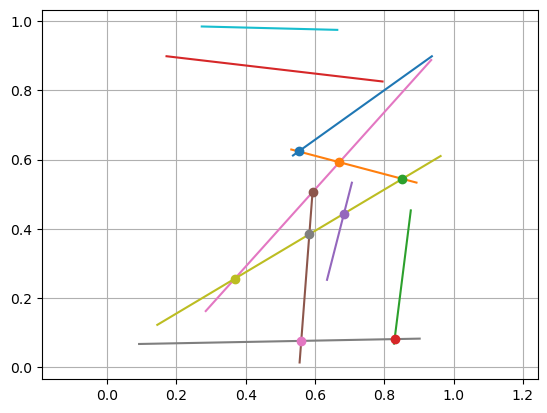# 12.2. Line-segment Intersections¶

A line-segment can be described by its two endpoints, $$p_1$$ and $$p_2$$, and denoted by $$p_1p_2$$. Note that the set of points on this segments can be expressed as $$p_1 + s(p_2-p_1)$$ for a parameter value $$s\in[0,1]$$.

To determine if $$p_1p_2$$ intersects another line-segment $$q_1q_2$$, we express the points on both segments in this way (with different parameters $$s,t$$), and set the points equal. This leads to the problem: Find $$s,t\in[0,1]$$ s.t.

$p_1 + s(p_2 - p_1) = q_1 + t(q_2 - q_1)$

which can be reorganized as a 2-by-2 linear system of equations for $$s,t$$:

$\begin{split} \begin{pmatrix} p_2 - p_1 & q_1 - q_2 \end{pmatrix} \begin{pmatrix} s \\ t \end{pmatrix} = \begin{pmatrix} q_1 - p_1 \end{pmatrix} \end{split}$

If the line-segments are parallel, the matrix is singular. For simplicity we simply say that they do not intersect in this case, but a better implementation would determine if they actually overlap.

We implement the method in the function below, where we have also included a tolerance $$\delta$$. This is useful to handle the case when the intersection is close to one of the endpoints, then $$\delta>0$$ would allow for a small positive overlap and $$\delta<0$$ a small negative overlap.

using LinearAlgebra
using PyPlot
function linesegment_intersect(p1, p2, q1, q2, δ)
A = [p2-p1 q1-q2]
b = q1-p1
if rank(A) == 2
st = A \ b
if all(-δ .≤ st .≤ 1 + δ)
pintersect = p1 + st * (p2 - p1)
return true, pintersect
else
return false, nothing
end
else
# Warning: parallel lines, should check if they actually overlap
return false, nothing
end
end

linesegment_intersect (generic function with 1 method)

# Example: n random lines, find and plot all intersections
n = 10
lines = [ [rand(2), rand(2)] for i = 1:n ]

# Plot all lines
for line in lines
plot([line, line], [line, line])
end

# Find all intersections - note that we only consider j>i to avoid checking
# the same 2 line-segments twice
for i = 1:n
for j = i+1:n
isect, pq = linesegment_intersect(lines[i], lines[i], lines[j], lines[j], 0)
if isect
plot(pq, pq, "o")
end
end
end

axis("equal"); grid(true);